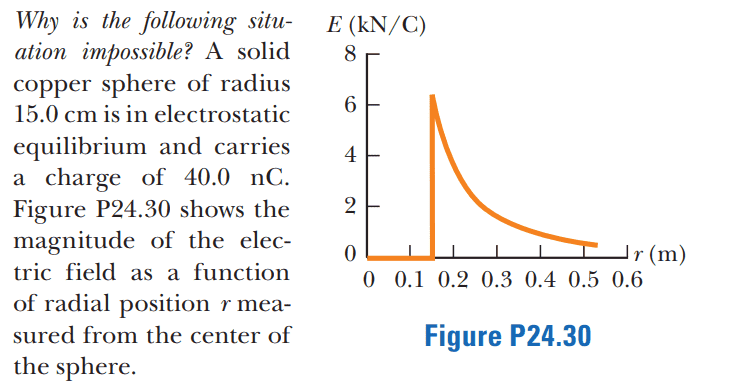# Electrostatic equilibrium problem

Callumnc1
Homework Statement:
Relevant Equations:
For this problem,Many thanks!

•anuttarasammyak

Homework Helper
Gold Member
What would be the magnitude of the electric field just outside the sphere?

•Callumnc1
Callumnc1
What would be the magnitude of the electric field just outside the sphere?
Thank you for your reply @kuruman ! The electric field of a sphere is equivalent to that of a point charge so ## E = \frac {40 \times 10^{-9}k_e}{0.15^2} ##

Many thanks!

Homework Helper
Gold Member
So why is the situation impossible?

•Callumnc1
Callumnc1
So why is the situation impossible?
Thanks for your reply @kuruman ! Thinking... Its got to me something to do with the electric field.

•